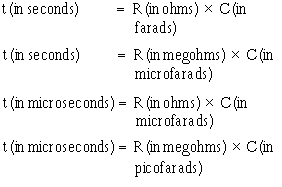Home   |  Schematics |  Products |  Tutorials  |  Datasheets  |  Robotics   |   Download    |   Link Exchange

 » Experiment » Calculator/Converters » Radio » Newsletter » Associations and Societies » Component Manufacturers

 Electronics Symentics A B C D E F G H I J K L M N O P Q R S T U V W X Y Z

 RC and LR Time Constants
 RC TIME CONSTANT The time required to charge a capacitor to 63 percent (actually 63.2 percent) of full charge or to discharge it to 37 percent (actually 36.8 percent) of its initial voltage is known as the TIME CONSTANT (TC) of the circuit. The charge and discharge curves of a capacitor are shown in figure 3-11. Note that the charge curve is like the curve in figure 3-9, graph (D), and the discharge curve like the curve in figure 3-9, graph (B). Figure 3-11. - RC time constant.The value of the time constant in seconds is equal to the product of the circuit resistance in ohms and the circuit capacitance in farads. The value of one time constant is expressed mathematically as t = RC. Some forms of this formula used in calculating RC time constants are:Q.14 What is the RC time constant of a series RC circuit that contains a 12-megohm resistor and a 12-microfarad capacitor? UNIVERSAL TIME CONSTANT CHART Because the impressed voltage and the values of R and C or R and L in a circuit are usually known, a UNIVERSAL TIME CONSTANT CHART (fig. 3-12) can be used to find the time constant of the circuit. Curve A is a plot of both capacitor voltage during charge and inductor current during growth. Curve B is a plot of both capacitor voltage during discharge and inductor current during decay. Figure 3-12. - Universal time constant chart for RC and RL circuit. The time scale (horizontal scale) is graduated in terms of the RC or L/R time constants so that the curves may be used for any value of R and C or L and R. The voltage and current scales (vertical scales) are graduated in terms of percentage of the maximum voltage or current so that the curves may be used for any value of voltage or current. If the time constant and the initial or final voltage for the circuit in question are known, the voltages across the various parts of the circuit can be obtained from the curves for any time after the switch is closed, either on charge or discharge. The same reasoning is true of the current in the circuit.